Half-Step Root Finding Method Introduction As another example application for the TI-89 or 92+, we will now demonstrate the creation of a simple root finding method. The purpose of this example is to demonstrate programming, plotting, lists and a few other things the TIs can do. What is the half-step method all about? In the half-step method, the user supplies an expression f(x), the variable x, a target x*, and a range [x1 .. x2] within which to locate the target value. The algorithm steps into that range, looking for the place where the sign of the function defined by f(x)-x* is opposite the one found at x=x1. When such a place is found, the algorithm backs up, reducing its step by half (hence the name), and continues to seek the sign change, but now moving with the reduced step. This process is repeated until an error tolerance (set by the user) is met. The following diagram describes the few first steps of such a process, working on the expression f(x)=x² with a target of x*=4, in the range [0 .. 5].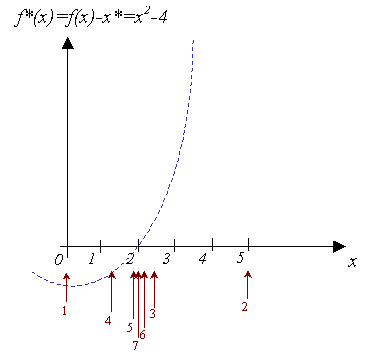As can be seen, this method is not one of the most efficient at all. However, its simplicity, both in application and concept make it one of my favorite methods, whenever complex problems are involved. Furthemore, for educational purposes this will serve us as a very good example. What are the advantages of this method? There are several useful features of this method. Simple concept, simple application Can be applied to almost any procedure which outputs a number and ultimately produces a smooth function† Requires a number of iterations which is almost the same for very different ranges‡ Follows a simple rule when more than one solution exists: If the range contains two of more solutions, the complimentary range will always converge to the other solutions, and never back to the one already found§ † In other words, the expression given to the algorithm can be a complete procedure in its own right, such as cases of the shooting method for ordinary differential equations, or very complex algorithms which have to be employed in order to obtain data points. It should be noted that very efficient methods, such as the Newton-Raphson method may require differentiation, which may be out of the question to calculate when such complex algorithms are involved. This makes the half-step method flexible and easy to adapt to the problem at hand. ‡ Even if not given a very close range in which to search for the solution (in cases when there is only one such solution), the method will converge in almost the same number of steps. For example, when the solution may be x*=5 then there will be a difference of only 3 or 4 iterations required, whether the given range is [0 .. 10], or [-100 .. 100]. This is a great advantage when there is very vague knowledge of the range in which the solution is. § Methods like Newton-Raphson or the secant method may sometimes not succeed in converging to other solutions until a very specific initial condition is found which leads to a different solution. This sometimes makes it difficult, for those working with the mentioned methods, to find other solutions, as it may take them quite a while to find the initial condition with which the method does not converge back to a solution already found. What are the disadvantages of this method? As mentioned, there are several disadvanteges: Slow convergence, relatively high number of iteraions required when other methods would converge in as little as 10 times less iterations. Sensitivity to expressions which do not cross the target value, but only touch it (e.g. absolute values, minimum and maximum problems) Sensitivity to expressions which are very fast-changing relative to the requested range, and change back to the initial sign very quickly.† † This situation may look something like this: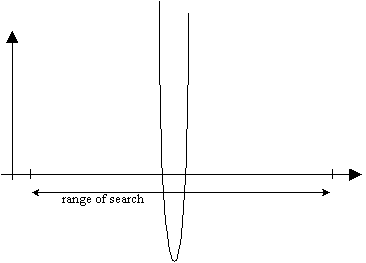In such cases the algorithm will eventually find the root (usually the leftmost), but it would take a while until it refines its step enough for it to catch the rapid sign change of the function. The algorithm for the half-step method The algorithm for the half-step method is simple. Here is a non-mathematical description of it: Variables: e - the expression                   target - the target                   x1 - lower boundary of search range                   x2 - upper boundary of search range                   tol - error tolerance within which to accept solution 1. Start at x = x1. 2. Set step size = distance between x1 and x2 3. Store the sign of the value   e(x) - target   in initsign. 4. Initialize the variable nosol with true if   | e(x) - target | > tol   is true. Othewise false. 5. While nosol is true do 6.       Add step size to x 7.       If x > x2 then we have reached the rightmost boundary x2 and need to get back to x1 8.           Reset x to x1 9.           Reduce step size by half refine the step so we could catch the change of sign 10.     End 11.     Update nosol with true if   | e(x) - target | > tol   is true. Othewise false. 12.     If sign( e(x) - target ) <> initsign and nosol is true then we have found a change of sign 13.         Substract step size from x take one step back 14.         Reduce step size by half now we would move forward only half the distance 15.     End 16. End ? Hmmm... What was that...? A "simple", "straightforward" method? ; ) Ans It may seem to be a bit cumbersome, but if you'll take a closer look, you'll notice that only the most basic mathematical operations are required: addition, absolute value and sign. It does not use differentiation, or expressions that include divisions (may cause problems when denominator approaches zero). Initial values are also not used, but only a range, which in most cases is faily easy to determine (and in any case note the fourth advantage mentioned above). This method is straightforward in the sense that it requires only elementary math, and has a logical, easy to follow principle with very little exceptions. The algorithm in TI-BASIC Input:   e - the expression            var - the independent variable            target - the target            x1 - lower boundary of search range            x2 - upper boundary of search range            tol - error tolerance within which to accept solution Output: x* - the value of var which brings e to target within the error tolerance tol Local Variables: step - holds the current step size                             initsign - holds the first sign of the expression  e(x) - target                             nosol - holds true if solution has been found, otherwise false.                             val - holds the value of  e(x) - target 1.    Local initsign, nosol, val 2.    expr(string(e | var = a)&">e(a)") turns the expression into a function of the given variable 3.    x1 > x 4.    x2 - x1 > step 5.    e(x) - target > val we save the result in a variable, so we won't have to calculate it a second time 6.    sign(val) > initsign 7.    abs(val) > tol > nosol this simply puts true or false into nosol 8.    While nosol 9.        x + step > x 10.       If x > x2 Then 11.           x1 > x 12.           step / 2 > step 13.       EndIf 14.       e(x) - target > val 15.       abs(val) > tol > nosol 16.       If sign(val) <> initsign and nosol Then 17.           x - step > step 18.           step / 2 > step 19.       EndIf 20.   EndWhile 21.   Return x The complete program Clearly, a few crucial aspects are missing from the last algorithm. Namely: a way to stop it through a maximum number of iterations, or when there is no solution in the range, and it tries to catch the change of sign by reducing the step without end. The following is the complete program, plus visual results display and a final graphing of the convergence process. Input:   e - the expression            var - the independent variable            target - the target            range - lower and upper boundary of search range in a list            tol - error tolerance within which to accept solution Output: x* - the value of var which brings e to target within the error tolerance tol             xlist - a list containing values the values of var through which the algorithm went.             ilist - a list containing a sequance of the iterations corresponding to the values in xlist.             (the last two output variables are only for our educational purposes) Local Variables: step - holds the current step size                           x - the current value of var                           initsign - holds the first sign of the expression  e(x) - target                           nosol - holds true if solution has been found, otherwise false.                           val - holds the value of  e(x) - target                           i, mi - counters for iterations and missed iterations number.                           maxi - holds the maximum number of iterations allowed                           maxmi - holds the maximum number of missed iterations allowed                           sgn - this variable will be a sign function, but which includes zero as positive 0.  halfstep(e, var, target, range, tol) 1.  Prgm 2.  Local initsign, nosol, val, i, mi, maxi, maxmi, sgn, step, x 3.  when(x >= 0, 1, -1) -> sgn(x) this makes a modified sign functions which includes a positive zero 5.  1 -> i 6.  0 -> mi 7.  50 -> maxi 9.  3 -> maxmi 10. expr(string(e | var = xyz)&">e(xyz)") turns the expression into a function of the given variable 11. range > x 12. range - range > step 13. e(x) - target > val we save the result in a variable, so we won't have to calculate it a second time 14. sgn(val) > initsign 15. abs(val) > tol > nosol this simply puts true or false into nosol 16. {x} -> xlist 17. While nosol and i < maxi and mi < maxmi 18.  x + step > x move forward one step size 19.  If x > range Then we have reached the rightmost boundary of the given range 20.   range > x reset x to leftmost point 21.   step / 2 > step refine step size 22.   mi + 1 -> mi update number of missed iterations 23.  EndIf 24.  x -> list[i+1] update x's list 25   e(x) - target > val 26.  abs(val) > tol > nosol 27.  If sign(val) /= initsign and nosol Then 28.   x - step > step move back one step size 29.   step / 2 > step refine step size 30.  EndIf 31.  i + 1 -> i update number of iterations 32. EndWhile 33. ClrIO 34. If i = maxi Then 35.  Disp "Max number of iterations" 36.  Disp "reached with "&string(var)&" = "&string(x) 37. ElseIf mi = maxmi Then 38.  Disp "Max number of missed iterations" 39.  Disp "reached with "&string(var)&" = "&string(x) 40. Else 41.  Disp "Ended with "&string(var)&" = "&string(x) 42.  Disp "Error = "&string( e(x) - target ) 43.  Disp "Iterations: "&string(i) 44. EndIf 45. Disp "" 46. Pause "Press [ENTER] ..." 47. If i>1 then 48.  seq(j, j, 1, i) -> ilist 49.  NewPlot 1, 1, ilist, xlist,,,,4 50.  ZoomData 51. Else 52.  DispHome 53. EndIf 54. EndPrgm For your convenience, you can download the program here: TI-89, TI-92+ A brief discussion of key features used in this program 1. Use of when( to define functions As shown in line 3, it is very convenient to use when( when a function operates on a conditional base. If we had written sgn( in full, we would have written something like this: sgn(x) Func  If x >= 0 Then   Return 1  Else   Return -1  EndIf EndFunc Obviously, when a single, simple condition is involved, it is much quicker to define the function using when( 2. Turning an expression + variable combination into function(variable) Sometimes we need the user to enter an expression and a variable to work with in that expression, such as in this example, or as used in many functions on the TIs (for example: d(expression, variable) ). In the absence of other options, we would have had to evaluate the expression with respect to variable, when variable has the value of value in the following way:                                                expression | variable = value For example, the program we've written halfstep( takes an expression e and a variable var. Normally, we would have had to write:   e | var = 2 -> b  every time we wanted to evaluate e when var is 2, and assign it to b. There is a better solution. If we could make a function e(var), then it would be much easier to write e(2), than all the above. The method is to write, in the general case, something like this:          expr(string(expression | variable = xyz)&">f(xyz)") This creates a function f(, which can be used instead of the longer combination. The variable xyz is just an intermidiate variable, and the funny name was chosen so as to make sure that no such variable already exists (that would simply return an error). The variable f can be local, or global (i.e. it can be declared for use inside the program only, with Local ..., or it can be made a variable that comtinued to exist after the program has finished). Here we've simply reused the variable e (line 10), so that it will be local and we wouldn't use memory on another variable (even local variables take memory...) 3. Assigning true of false directly without using If .. Then ... Else ... EndIf In line 15 we use a short way to assign true or false to the variable nosol. The long, formal way would have been to write: If abs(val) > tol Then  true -> nosol Else  false -> nosol EndIf As before, the short way is shorter, and not much remains to be said. 4. Appending to a list In accordance with tip 3.10 in Doug Burkett's The TipList, it is faster to append to the list, rather than to use the function augment(. This is implemented here in line 24. Notice that the first value of x is already stored in the list in line 16, and so we begin appending from index 2, and this is the reason for the i + 1. If the list is started from blank, then we begin from index 1, as this little example shows: {} -> list For i, 1, 5  i -> list[i] EndFor This creates the list {1, 2, 3, 4, 5}. Note that as error will occur if the program tries to append to an indexs greater than the last + 1. 5. Plotting a series of points (x, y) from a program The command which defines a plot is called NewPlot, and we use it in line 49. The general syntax is:                NewPlot n, type, xlist, ylist,,,, mark No, the four commas are not a typo. The reason is that there can appear other options in between, which we ignore here (please consult your manual for a complete description of how to use NewPlot). n is the plot number (1 for Plot1, 2 for Plot2, etc.) type is 1 or 2, for scatter and xyline respectively. xlist contains the x values ylist contains the corresponding y values mark is 1 for box, 2 for cross, 3 for plus, 4 for square and 5 for dot. The only catch is this: the lists have to be global variables! In other words, it wouldn't work to write:                                                 NewPlot 1, 1, {1, 2, 3}, {1, 2, 3},,,, 4 Though the lists are lists, the are not variables,and so this would create and error. Think about it this way: with the command NewPlot you are asking to define a plot using two lists. However, this means that the plot has to be available later, while a quick look at the plot definition dialog box shows us that it does not store the lists itself (the values themselves), but only the lists names.This is the reason the variable xlist is not a local variable, and likewise ilist isn't, in line 48. After this command, which only defines the plot, the TI does not automatically switch to the graph screen. We do this manually with the ZoomData command in line 50, which adjusts the window limits to those of the data points. A demonstration of the Half-Step program We will use a simple example here: finding when f(x)=x² is 4, with an error tolerance of 0.001. We first start with a 'good' range: [0 .. 5].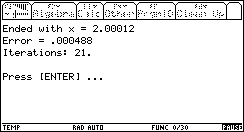Note: use Diamond+ENTER to get a floating point result.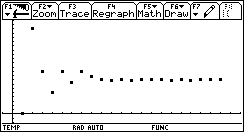Quickly tracing the values with F3: Trace, shows us the progress of the algorithm. The last value is, of course, the first value which is within the error tolerance tol.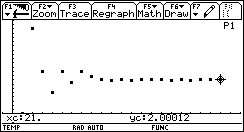We can try the same thing with a 'bad' range: [10 .. 20]. The algorithms prgress would look like this: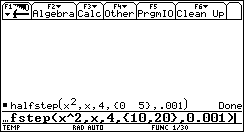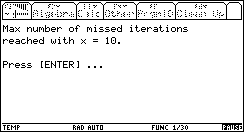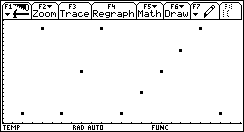The explanation is obvious: the algorithm couldn't find a sign change between 10 and 20, so it decreases its step size again and again, until the entire range is missed for the 3rd time (this is maxmi). A negative range [-5 .. 0] will give the other solution: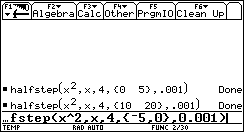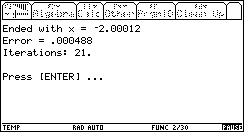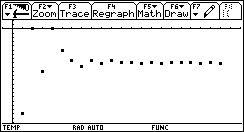But what happens when we have absolutely no idea where the solution is? Let's take an exagerrated range of [-100 .. 100]. This is what happens: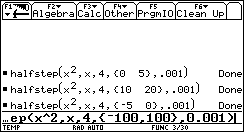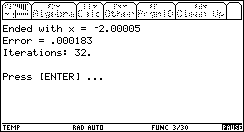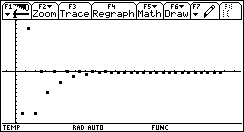As expected, the algorithm converged to the negative solution (because it is the first, whereas the positive is the second, in terms of the algorithm's progress). Notice the price for our exaggerated large range of [-100 .. 100]: only 11 more iterations! 50% more iteartions than when we gave the range [-5 .. 0] ! Considering the fact that the range was now 40 times bigger than [-5 .. 0], this is remarkable. Let's try an even more exagerrated range: [-1000 .. 1000]. How many more iterations will it take this time (the range will be 400 times larger than [-5 .. 0])?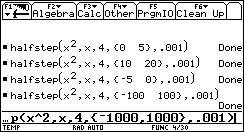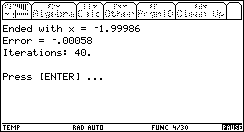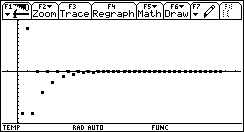It now took 40 iteartions to converge to a solution! This is twice as much as with [-5 .. 0], when the range is 400 times larger! Conclusions Obviously, this method is not the best, when it comes to root finding. We have seen its slowness, even when the range boundaries ware very close to the solution. On the other hand, we have seen it's speed, when the range boundaries are very far from the solution. This example is long enough already, so we will not discuss other, more delicate cases now. For the educational purposes, I rest my case :) Congratulations! You have completed this task! ______________________________________________________________________________________ Created by Andrew Cacovean, March 16, 2002